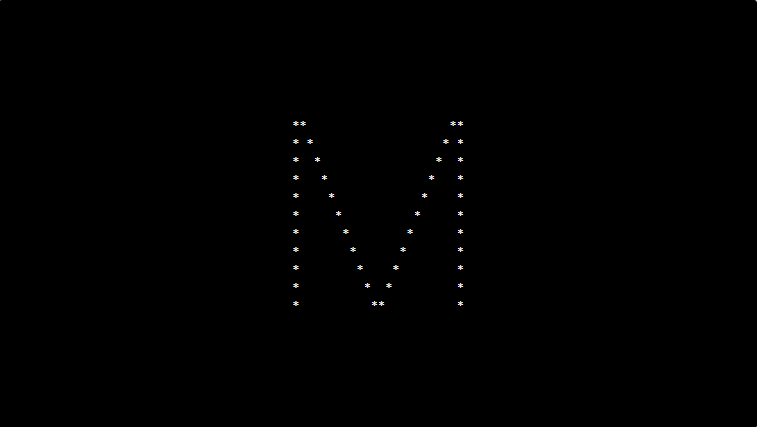# PHP program to print alphabet pattern M

In this article write a PHP program to print alphabet pattern M. This Program first takes the numbers of rows and then prints pattern using nested for loops.

## PHP program to print alphabet pattern M

```<?php
echo "<pre>";
\$j = 22;
for (\$row = 0; \$row <= 10; \$row++) {
for (\$col = 0; \$col <= 22; \$col++) {
if (\$col == \$j) {
echo "*";
\$j = \$j - 1;
}
if (\$col == 0 OR \$col == 22 OR \$row == \$col - 1) {
echo "*";
} else {
echo "&nbsp;";
}
}
echo "<br/>";
}
echo "</pre>";
?>
```

### Output:1 Comment
1. kiran says

print this pattern
* * * * *
* * * *
* *
write a php code this pattern display?